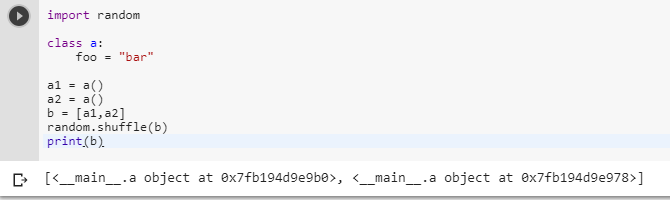2 views
in Python

I have a list of objects in Python and I want to shuffle them. I thought I could use the random.shuffle method, but this seems to fail when the list is of objects. Is there a method for shuffling object or another way around this?

import random

class a:

foo = "bar"

a1= a()

a2= a()

b = [a1,a2]

print random.shuffle(b)

This will fail.

by (106k points)
• In My code random.shuffle works, here's an example regarding that:

from random import shuffle

x = [[i] for i in range(10)]

shuffle(x)

print x

• You should also keep this thing in mind that shuffle does not return any value

• For your code, you just need to replace some line of code which I am doing down here:-

import random

class a:

foo = "bar"

a1= a()

a2= a()

b = [a1,a2]

random.shuffle(b)

print(b)